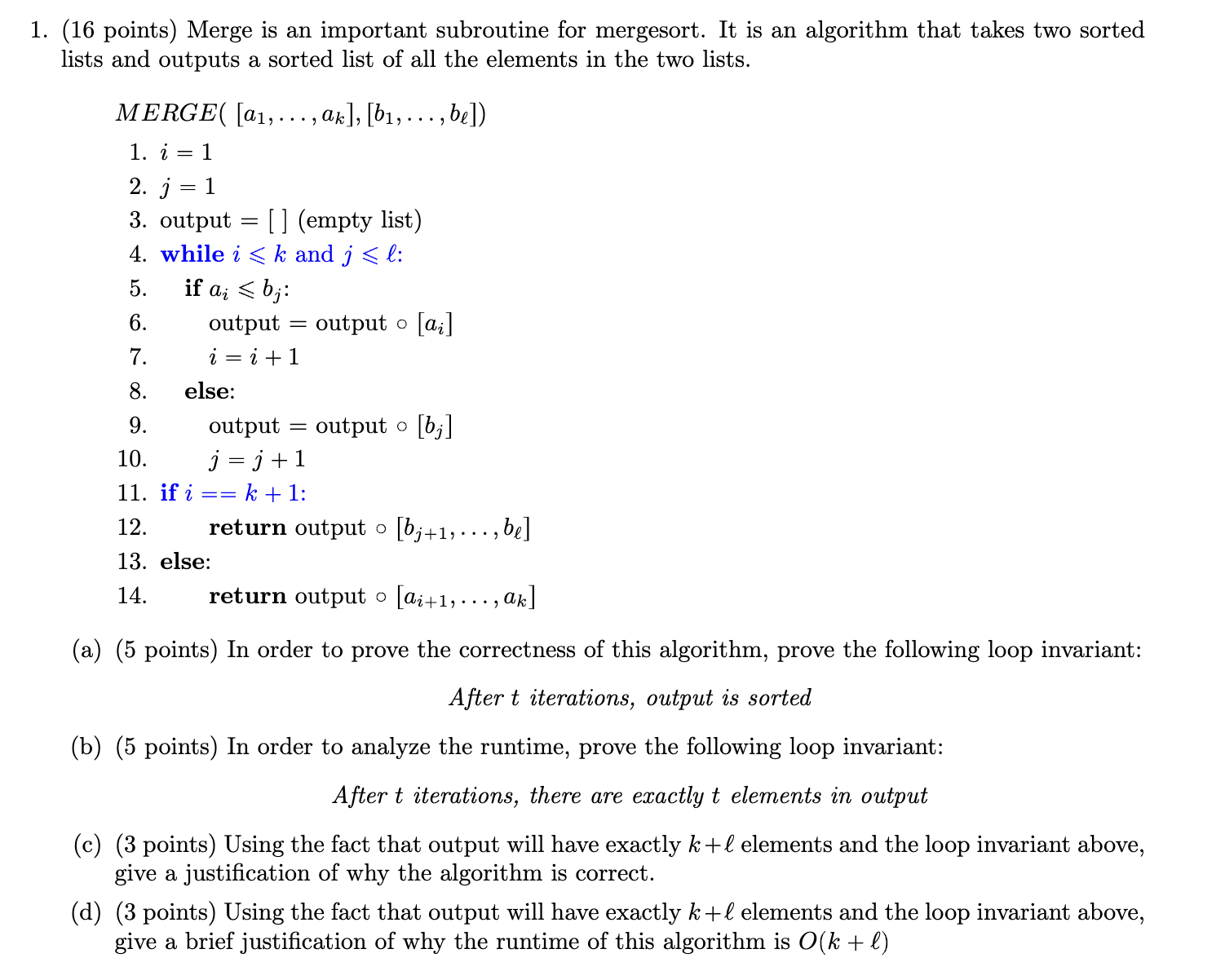Home / Expert Answers / Computer Science / 1-16-points-merge-is-an-important-subroutine-for-mergesort-it-is-an-algorithm-that-takes-two-so-pa187

# (Solved): 1. (16 points) Merge is an important subroutine for mergesort. It is an algorithm that takes two so ...1. (16 points) Merge is an important subroutine for mergesort. It is an algorithm that takes two sorted lists and outputs a sorted list of all the elements in the two lists. 1. 2. 3. output (empty list) 4. while and : 5. if : 6. output output 7. 8. else: 9. output output 10. 11. if : 12. return output 13. else: 14. return output (a) (5 points) In order to prove the correctness of this algorithm, prove the following loop invariant: After iterations, output is sorted (b) (5 points) In order to analyze the runtime, prove the following loop invariant: After iterations, there are exactly elements in output (c) (3 points) Using the fact that output will have exactly elements and the loop invariant above, give a justification of why the algorithm is correct. (d) (3 points) Using the fact that output will have exactly elements and the loop invariant above, give a brief justification of why the runtime of this algorithm is

We have an Answer from Expert

The question is about Merge subroutine used in the MergeSort algorithm. Merge takes two sorted lists as input and merges them into a single sorted list

By addressing each part of the question, you will provide a thorough explanition of the correctness and runtime analysis of the Merge algorithm used in mergesort.
We have an Answer from Expert